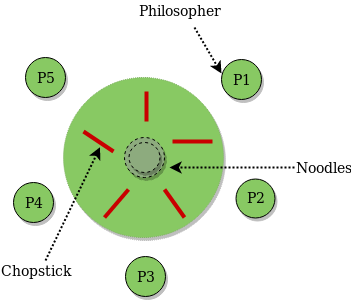# Dining Philosopher Problem Using Semaphores

• Difficulty Level : Medium
• Last Updated : 18 Aug, 2021

Prerequisite – Process Synchronization, Semaphores, Dining-Philosophers Solution Using Monitors
The Dining Philosopher Problem – The Dining Philosopher Problem states that K philosophers seated around a circular table with one chopstick between each pair of philosophers. There is one chopstick between each philosopher. A philosopher may eat if he can pick up the two chopsticks adjacent to him. One chopstick may be picked up by any one of its adjacent followers but not both.Semaphore Solution to Dining Philosopher –
Each philosopher is represented by the following pseudocode:

```process P[i]
while true do
{  THINK;
PICKUP(CHOPSTICK[i], CHOPSTICK[i+1 mod 5]);
EAT;
PUTDOWN(CHOPSTICK[i], CHOPSTICK[i+1 mod 5])
}```

There are three states of the philosopher: THINKING, HUNGRY, and EATING. Here there are two semaphores: Mutex and a semaphore array for the philosophers. Mutex is used such that no two philosophers may access the pickup or putdown at the same time. The array is used to control the behavior of each philosopher. But, semaphores can result in deadlock due to programming errors.
Code –

## C

 `#include ` `#include ` `#include `   `#define N 5` `#define THINKING 2` `#define HUNGRY 1` `#define EATING 0` `#define LEFT (phnum + 4) % N` `#define RIGHT (phnum + 1) % N`   `int` `state[N];` `int` `phil[N] = { 0, 1, 2, 3, 4 };`   `sem_t mutex;` `sem_t S[N];`   `void` `test(``int` `phnum)` `{` `    ``if` `(state[phnum] == HUNGRY` `        ``&& state[LEFT] != EATING` `        ``&& state[RIGHT] != EATING) {` `        ``// state that eating` `        ``state[phnum] = EATING;`   `        ``sleep(2);`   `        ``printf``(``"Philosopher %d takes fork %d and %d\n"``,` `                      ``phnum + 1, LEFT + 1, phnum + 1);`   `        ``printf``(``"Philosopher %d is Eating\n"``, phnum + 1);`   `        ``// sem_post(&S[phnum]) has no effect` `        ``// during takefork` `        ``// used to wake up hungry philosophers` `        ``// during putfork` `        ``sem_post(&S[phnum]);` `    ``}` `}`   `// take up chopsticks` `void` `take_fork(``int` `phnum)` `{`   `    ``sem_wait(&mutex);`   `    ``// state that hungry` `    ``state[phnum] = HUNGRY;`   `    ``printf``(``"Philosopher %d is Hungry\n"``, phnum + 1);`   `    ``// eat if neighbours are not eating` `    ``test(phnum);`   `    ``sem_post(&mutex);`   `    ``// if unable to eat wait to be signalled` `    ``sem_wait(&S[phnum]);`   `    ``sleep(1);` `}`   `// put down chopsticks` `void` `put_fork(``int` `phnum)` `{`   `    ``sem_wait(&mutex);`   `    ``// state that thinking` `    ``state[phnum] = THINKING;`   `    ``printf``(``"Philosopher %d putting fork %d and %d down\n"``,` `           ``phnum + 1, LEFT + 1, phnum + 1);` `    ``printf``(``"Philosopher %d is thinking\n"``, phnum + 1);`   `    ``test(LEFT);` `    ``test(RIGHT);`   `    ``sem_post(&mutex);` `}`   `void``* philosopher(``void``* num)` `{`   `    ``while` `(1) {`   `        ``int``* i = num;`   `        ``sleep(1);`   `        ``take_fork(*i);`   `        ``sleep(0);`   `        ``put_fork(*i);` `    ``}` `}`   `int` `main()` `{`   `    ``int` `i;` `    ``pthread_t thread_id[N];`   `    ``// initialize the semaphores` `    ``sem_init(&mutex, 0, 1);`   `    ``for` `(i = 0; i < N; i++)`   `        ``sem_init(&S[i], 0, 0);`   `    ``for` `(i = 0; i < N; i++) {`   `        ``// create philosopher processes` `        ``pthread_create(&thread_id[i], NULL,` `                       ``philosopher, &phil[i]);`   `        ``printf``(``"Philosopher %d is thinking\n"``, i + 1);` `    ``}`   `    ``for` `(i = 0; i < N; i++)`   `        ``pthread_join(thread_id[i], NULL);` `}`

Note – The below program may compile only with C compilers with semaphore and pthread library.
References –
Solution of Dining Philosophers – cs.gordon.edu
Solution of Dining Philosophers – cs.indiana.edu

My Personal Notes arrow_drop_up
Recommended Articles
Page :